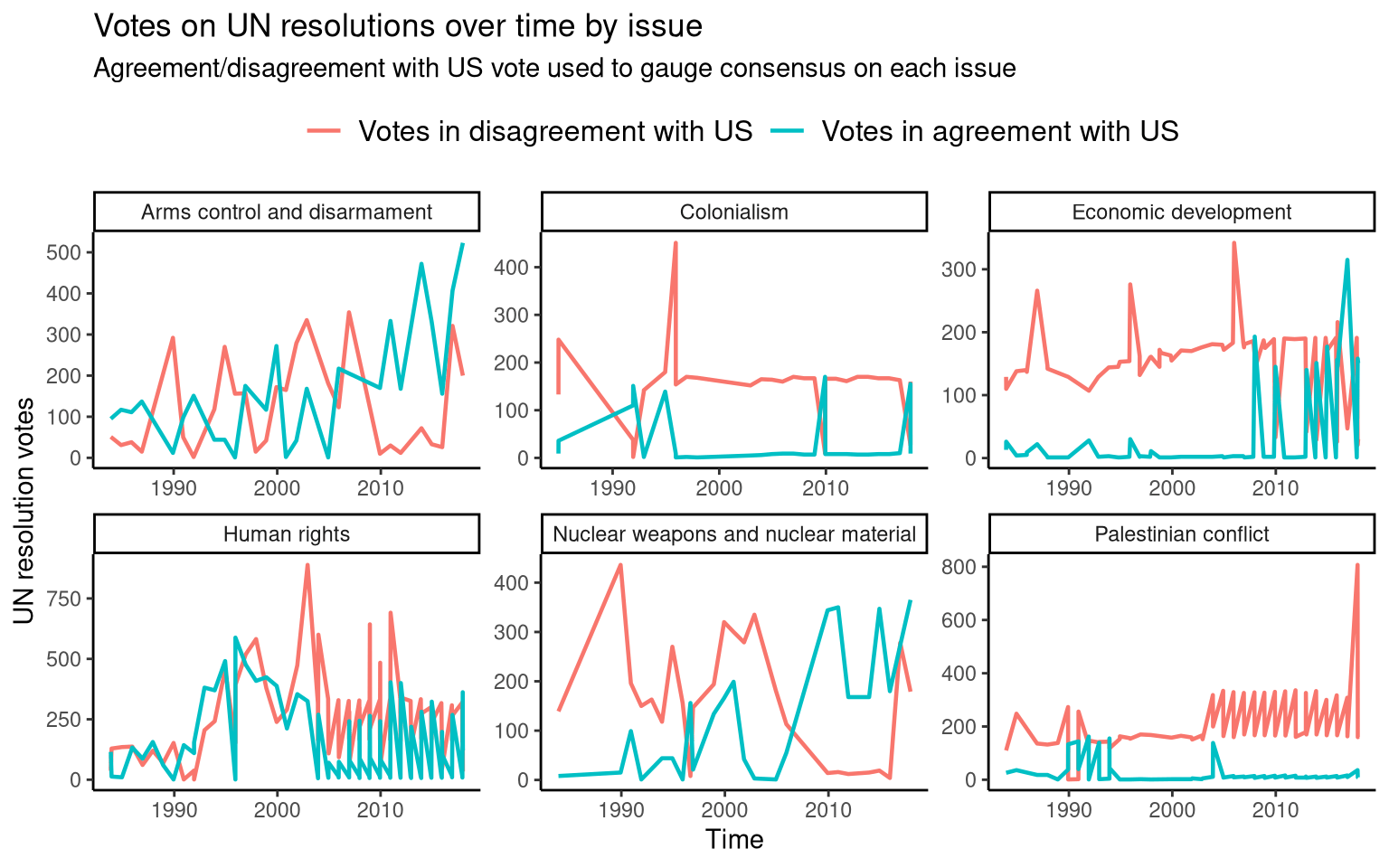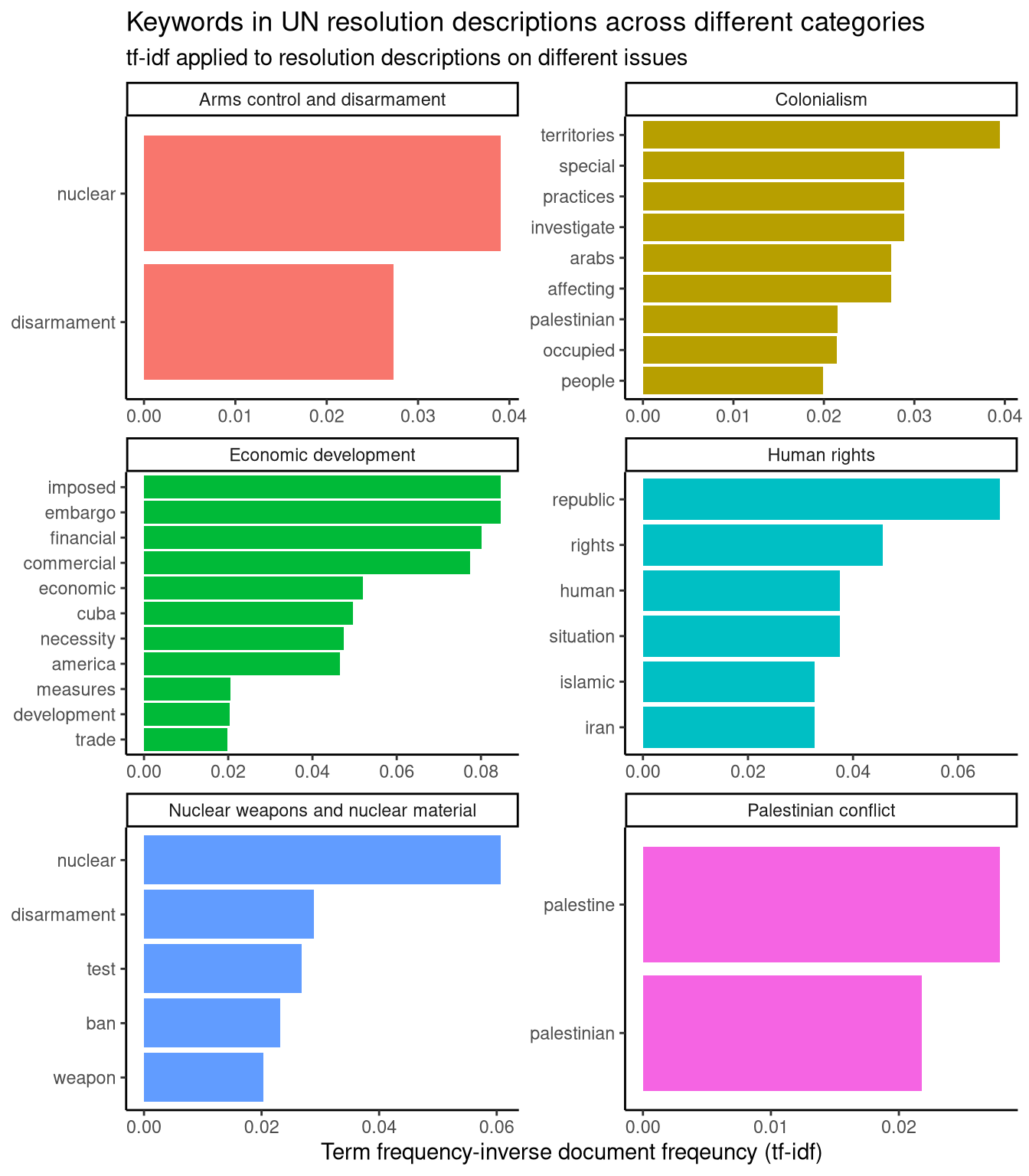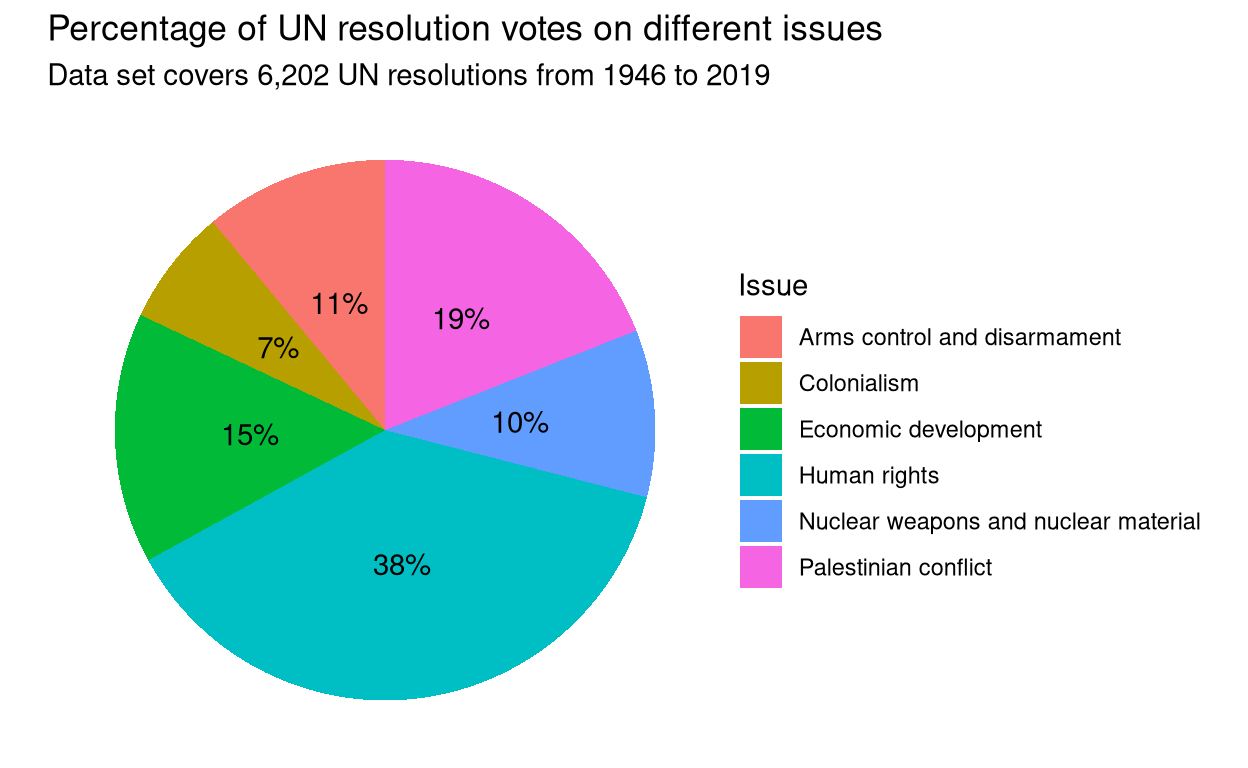Want to share your content on R-bloggers? click here if you have a blog, or here if you don't.

## Setup and data preparation

Loading the `R` libraries and data set.

```# Loading libraries
library(forcats)
library(tidytext)
library(tidyverse)
library(tidytuesdayR)

Wrangling data for visualisation.

```# Extracting US votes on each UN resolution
filter(country_code == "US") %>%
select(rcid, vote)

# Combining US votes and resolution issues with the "roll_calls" data set for
importantvote == 1)
by = "rcid")
by = "rcid")
filter(country != "United States") %>%
mutate(agree_with_US = vote == US_vote)
 "FALSE" "TRUE"
tt\$issues, by = "rcid")
# A tibble: 88,144 x 16
rcid session importantvote date       unres  amend  para short
<dbl>   <dbl>         <dbl> <date>     <chr>  <dbl> <dbl> <chr>
1  2491      38             1 1983-10-04 R/38/3     0     0 KAMPUCHEA
2  2491      38             1 1983-10-04 R/38/3     0     0 KAMPUCHEA
3  2491      38             1 1983-10-04 R/38/3     0     0 KAMPUCHEA
4  2491      38             1 1983-10-04 R/38/3     0     0 KAMPUCHEA
5  2491      38             1 1983-10-04 R/38/3     0     0 KAMPUCHEA
6  2491      38             1 1983-10-04 R/38/3     0     0 KAMPUCHEA
7  2491      38             1 1983-10-04 R/38/3     0     0 KAMPUCHEA
8  2491      38             1 1983-10-04 R/38/3     0     0 KAMPUCHEA
9  2491      38             1 1983-10-04 R/38/3     0     0 KAMPUCHEA
10  2491      38             1 1983-10-04 R/38/3     0     0 KAMPUCHEA
# … with 88,134 more rows, and 8 more variables: descr <chr>,
#   country <chr>, country_code <chr>, vote <chr>, US_vote <chr>,
#   agree_with_US <fct>, short_name <chr>, issue <chr>
# Counting the number of votes for each country that coincide with the US vote
# on each resolution
group_by(agree_with_US) %>%
select(country, agree_with_US) %>%
count(agree_with_US, country, sort = TRUE)
agree_with_US_count\$country <- as.factor(agree_with_US_count\$country)

# Counting the number of votes that are the same/different as the US vote on
# UN resolutions in different categories
filter(!is.na(issue)) %>%
select(date, agree_with_US, issue) %>%
group_by(date, issue) %>%
count(agree_with_US)

# Calculating term frequency-inverse document frequency (tf-idf) for the
# descriptions of UN resolutions across different issues
filter(!is.na(issue)) %>%
select(issue, descr) %>%
unnest_tokens(word, descr) %>%
count(issue, word, sort = TRUE)
issue_descr_words\$issue <- as.factor(issue_descr_words\$issue)
# Removing "stop words" from resolution descriptions (e.g. "the", "and", "of")
issue_descr_words <- issue_descr_words %>%
anti_join(stop_words, by = "word")
total_words <- issue_descr_words %>%
group_by(issue) %>%
summarise(total = sum(n))
issue_descr_words <- left_join(issue_descr_words, total_words, by = "issue")
issue_descr_words <- issue_descr_words %>%
bind_tf_idf(word, issue, n)```

## Plotting the countries that have agreed or disagreed with the most US votes

One of the variables in this data set is `importantvote`, which is used to record whether or not each United Nations (UN) resolution is classified as important by the United States (US) State Department. This is taken from its “Voting Practices in the United Nations” report. This variable is used to select UN resolutions important to the US. For these important resolutions, the vote of each country is compared with the US vote. The number of times each country votes with or against the US is counted; the countries that have agreed/disagreed on the most resolutions are plotted below.

```# Plotting countries that have agreed/disagreed with the most US votes
agree_with_US_count %>%
ggplot(aes(n, fct_reorder(country, n), fill = agree_with_US)) +
geom_col(show.legend = FALSE) +
facet_wrap(~agree_with_US, ncol = 2, scales = "free") +
theme_classic() +
labs(x = "Votes on important UN resolutions", y = "Countries",
title = "Votes on important United Nations (UN) resolutions",
subtitle = "Resolution importance classified by the United States (US) State Department")```## Plotting votes on UN resolutions on different issues

The comparison of each UN resolution vote with the US vote is also used in this plot. In this case, it is used to gauge the amount of consensus on each issue.

```# Plotting votes on different categories of UN resolutions over time
ggplot(aes(x = date, y = n, group = agree_with_US, colour = agree_with_US)) +
geom_line(size = 0.8) +
facet_wrap(~issue, scales = "free") +
theme_classic() +
theme(legend.position = "top") +
theme(legend.text = element_text(size = 12)) +
labs(x = "Time", y = "UN resolution votes",
title = "Votes on UN resolutions over time by issue",
subtitle = "Agreement/disagreement with US vote used to gauge consensus on each issue",
colour = NULL)```## Plotting keywords in UN resolution descriptions

The different issues covered by each UN resolution is taken directly from the data set (`tt\$issues`). In this section, significant words used to describe UN resolutions on each issue are plotted. This is done by…

• taking the UN resolution descriptions in `tt\$roll_calls\$descrl` as a corpus
• splitting that corpus into a document for each issue in `tt\$issues`
• calculating tf-idf to find significant words used to describe UN resolutions on each issue

These keywords outline which UN resolution issues pertain to different countries. For example, resolutions on “Human rights” frequently use the words “situation” and “Iran”; resolutions on “Economic development” frequently use the words “embargo” and “Cuba”; resolutions on “Colonialism” frequently use the words “territories” and “Palestinian”.

```# Plotting keywords in UN resolution descriptions
issue_descr_words %>%
group_by("issue") %>%
slice_max(tf_idf, n = 35) %>%
ungroup() %>%
ggplot(aes(tf_idf, fct_reorder(word, tf_idf), fill = issue)) +
geom_col(show.legend = FALSE) +
facet_wrap(~issue, ncol = 2, scales = "free") +
labs(x = "tf-idf", y = NULL) +
theme_classic() +
labs(x = "Term frequency-inverse document freqeuncy (tf-idf)",
title = "Keywords in UN resolution descriptions across different categories",
subtitle = "tf-idf applied to resolution descriptions on different issues")```## Plotting the percentage of UN votes on each issue in data set

This pie chart illustrates the percentage of UN votes on each issue in the data set.

```# Plotting percentage of votes in each UN resolution category
filter(!is.na(issue)) %>%
select(issue) %>%
group_by(issue) %>%
count(issue) %>%
ungroup() %>%
mutate(perc = round(n/sum(n) * 100)) %>%
ggplot(aes(x = "", y = perc, fill = issue)) +
geom_bar(stat="identity", width=1) +
coord_polar("y", start=0) +
theme_void() +
geom_text(aes(label = paste(perc, "%", sep = "")), position = position_stack(vjust=0.5)) +
labs(title = "Percentage of UN resolution votes on different issues",
subtitle = "Data set covers 6,202 UN resolutions from 1946 to 2019",
fill = "Issue")```